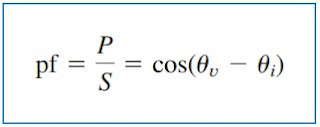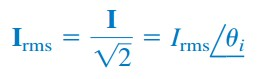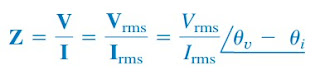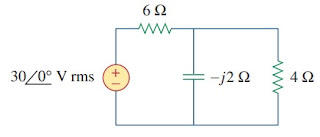omG8HI9LSKFW6lhCj8prTs0Z6lUhdOT9Jhi1Sf4m

# Power Factor Formula and Apparent Power for AC Circuits

Power factor is the ratio of working power. This term is often mentioned in the electrical industry, but do you know what it means?
Make sure to read what is ac circuit first.

## Apparent Power and Power Factor

We saw that if the voltage and current at the terminals of a circuit are(1)
or, in phasor form, VVm∠θv and I = Im∠θi, the average power is(2)
we saw that(3)
We have added a new term to the equation :(4)
The average power is a product of two terms. The product VrmsIrms is known as the apparent power S. The factor cos(θv - θi) is called the power factor (pf).
The apparent power (in VA) is the product of the rms values of voltage and current.
The apparent power is so-called because it seems apparent that the power should be the voltage-current product, by analogy with dc resistive circuits. It is measured in volt-amperes or VA to distinguish it from the average or real power, which is measured in watts. The power factor is dimensionless since it is the ratio of the average power to the apparent power,(5)
The angle θv - θi is called the power factor angle since it is the angle whose cosine is the power factor. The power factor angle is equal to the load impedance if V is the voltage across the load and I is the current through it. This is evident from the fact that(6)
Alternatively, since(7a)
and(7b)
the impedance is(8)
The power factor is the cosine of the phase difference between voltage and current. It is also the cosine of the angle of the load impedance.
From Equation.(5), the power factor may be seen as that factor by which the apparent power must be multiplied to obtain the real or average power. The value of pf ranges between zero and unity.

For a purely resistive load, the voltage and current are in phase, so that θv - θi = 0 and pf = 1. This implies that the apparent power is equal to the average power.

For a purely reactive load, θv - θi = ± 90o and pf = 0. In this case, the average power is zero. In between these two extreme cases, pf is said to be leading or lagging. Leading power factor means that the current leads voltage, which implies a capacitive load.

Lagging power factor means that current lags voltage, implying an inductive load. Power factor affects the electric bills consumers pay the electric utility companies.

## Apparent Power and Power Factor Examples

For better understanding let us review examples below :
1. A series-connected load draws a current i(t) = 4 cos(100π t + 10o) A when the applied voltage is v(t) = 120 cos(100π t - 20o) V. Find the apparent power and the power factor of the load. Determine the element values that form the series-connected load.
Solution :
The apparent power is
The power factor is
The pf is leading because the current leads the voltage. The pf may also be obtained from the load impedance.
The load impedance Z can be modelled by a 25.98 Ω resistor in series with a capacitor with
or

2. Determine the power factor of the entire circuit of Figure.(1) as seen by the source. Calculate the average power delivered by the source.Figure 1
Solution :
The total impedance is
The power factor is
since the impedance is capacitive. The rms value of the current is
The average power supplied by the source is
or
where R is the resistive part of Z.

Have you understood what is power factor? Don't forget to share and subscribe! Happy learning!
Reference:  Fundamentals of electric circuits by Charles K. Alexander and Matthew N. O. Sadiku

Untuk Bahasa Indonesia baca Pengertian Daya Semu dan Rumus Faktor Daya.
Related Posts
SHARE